# RD Sharma Solutions for Class 9 Maths Chapter 16 Circles Exercise VSAQs

RD Sharma class 9 Mathematics chapter 16 exercise VSAQs solutions for circles are provided here. In this exercise, students will revise all the concepts listed in this chapter. Thus, here we have our set of RD Sharma solutions for class 9 to help students in their endeavor to have a better understanding of circles.

## Download PDF of RD Sharma Solutions for Class 9 Maths Chapter 16 Circles Exercise VSAQs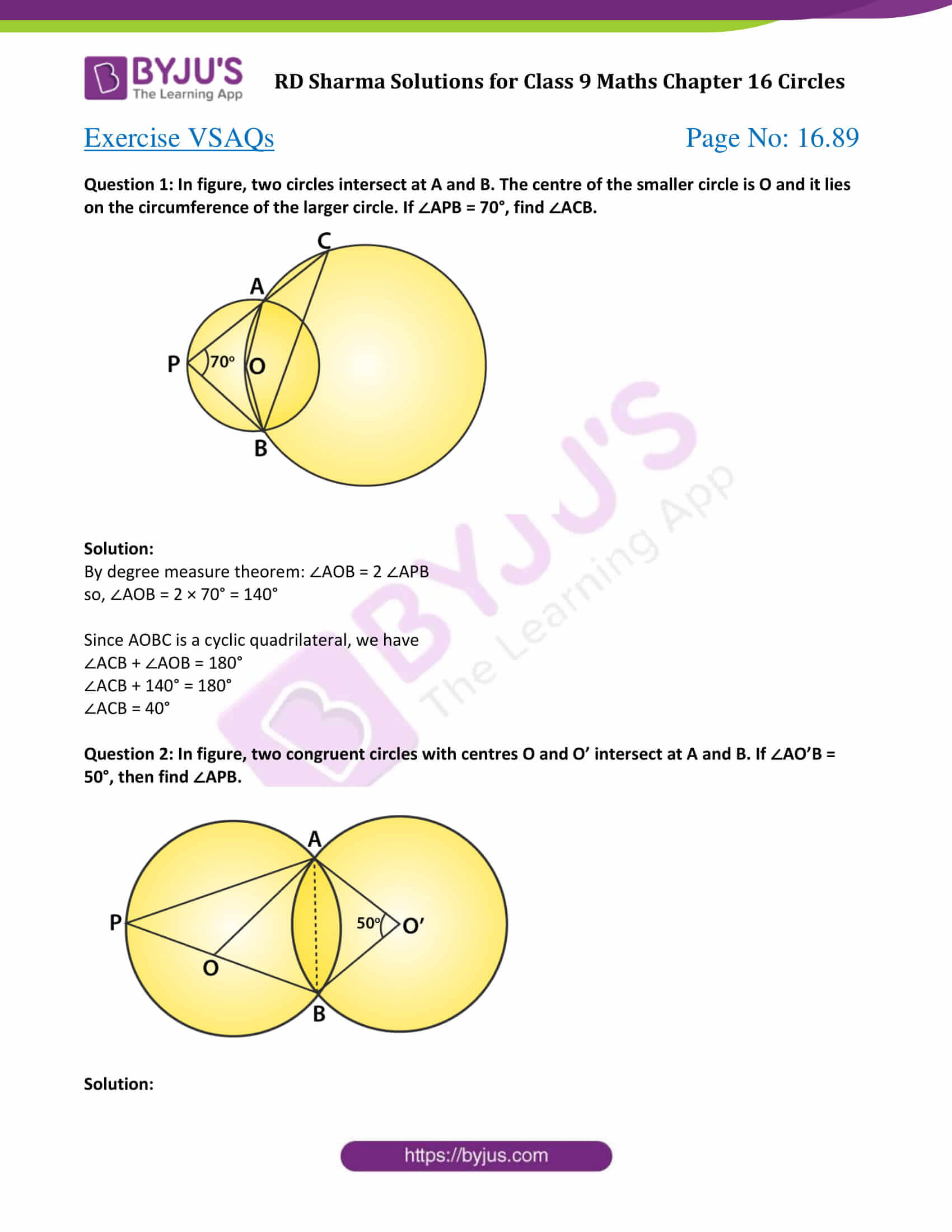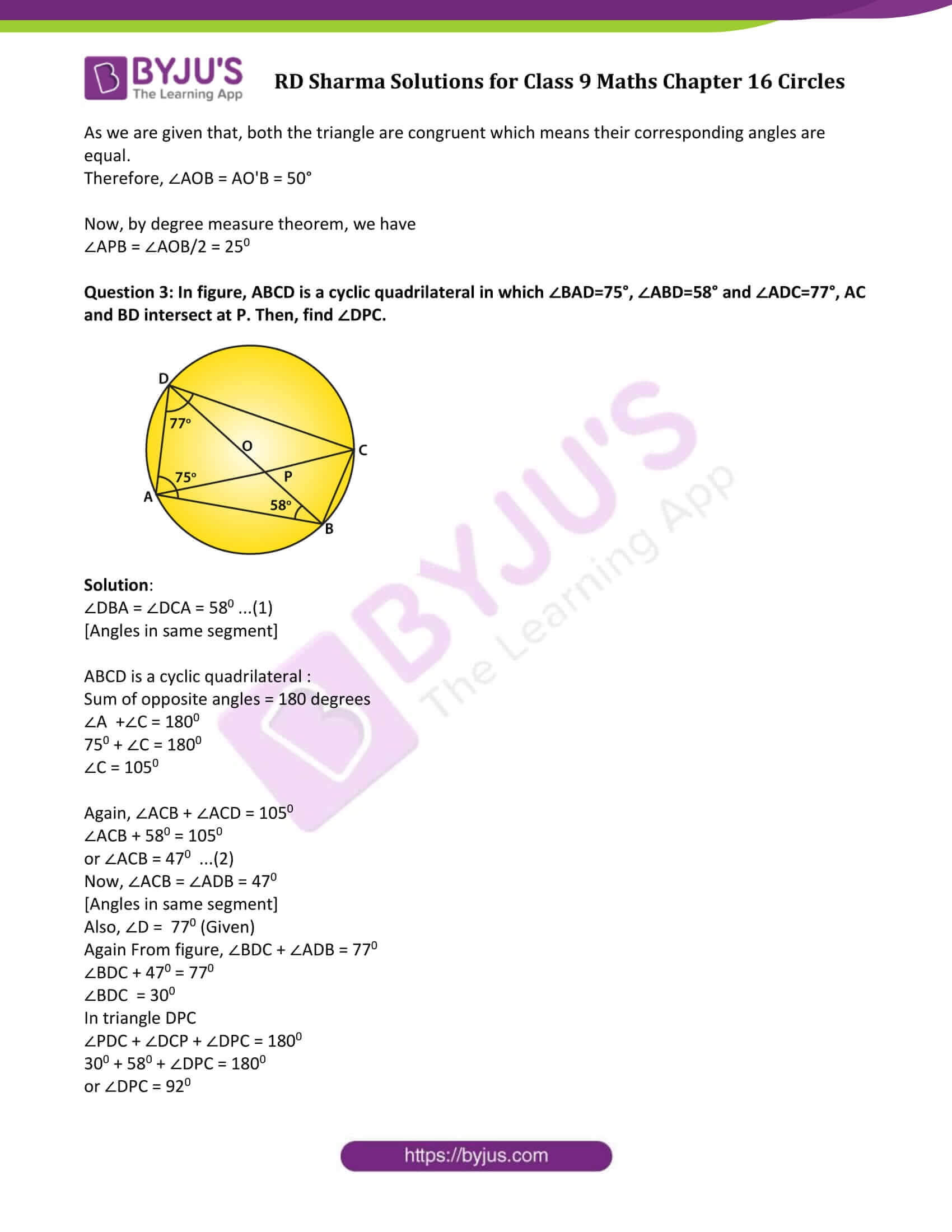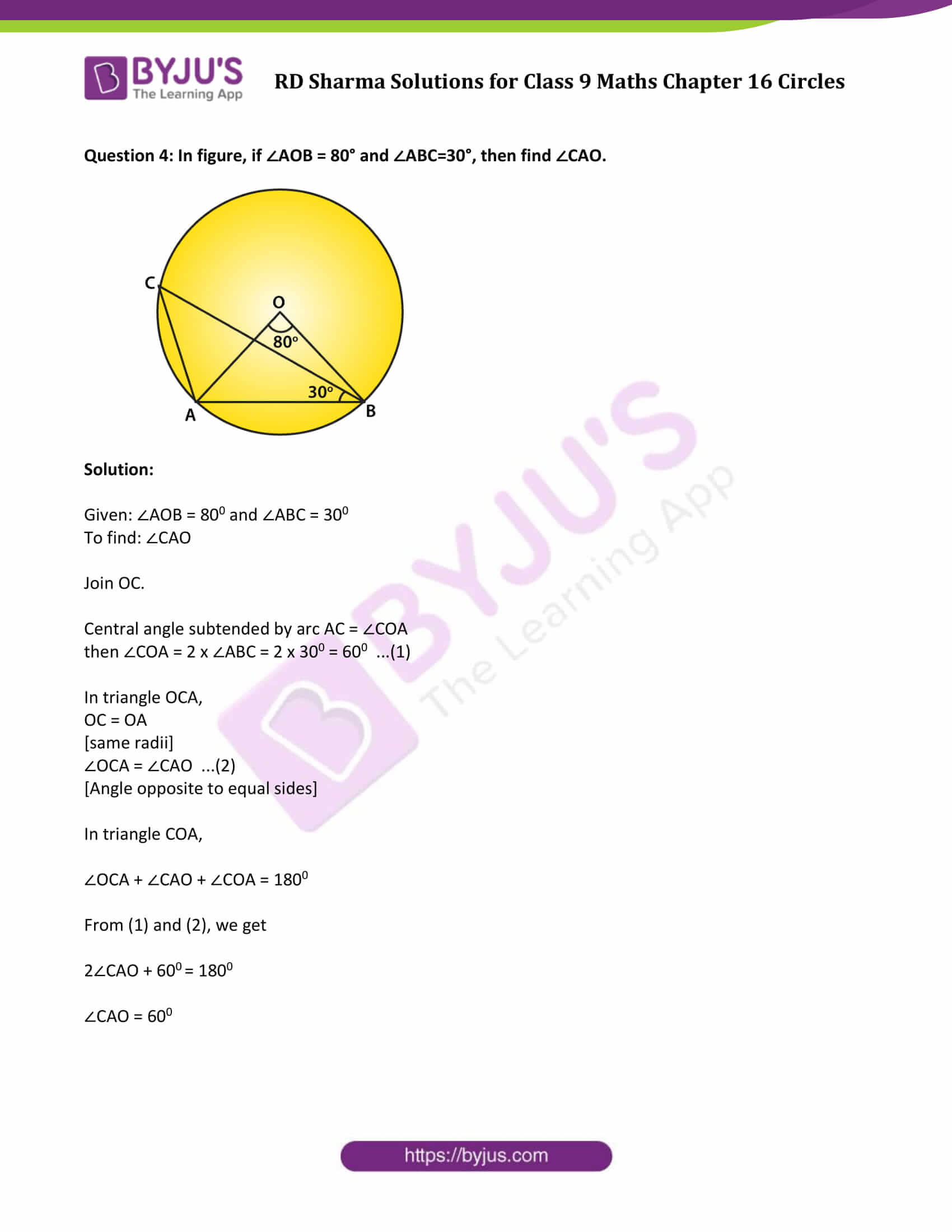### Access Answers to Maths RD Sharma Solutions for Class 9 Chapter 16 Circles Exercise VSAQs Page number 16.89

Question 1: In figure, two circles intersect at A and B. The centre of the smaller circle is O and it lies on the circumference of the larger circle. If ∠APB = 70°, find ∠ACB.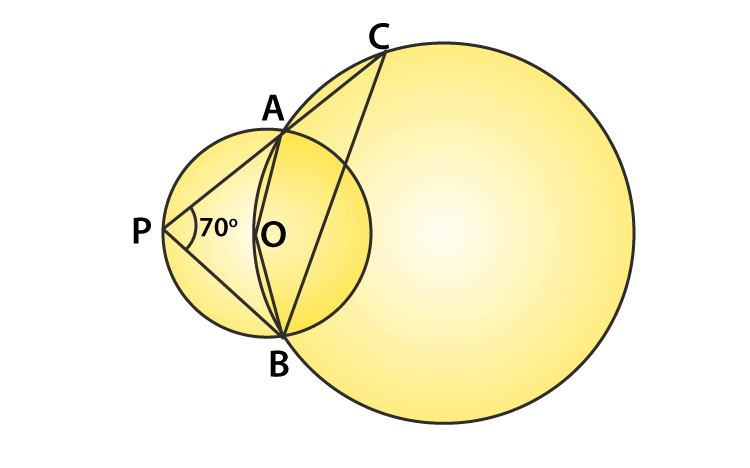Solution:

By degree measure theorem: ∠AOB = 2 ∠APB

so, ∠AOB = 2 × 70° = 140°

Since AOBC is a cyclic quadrilateral, we have

∠ACB + ∠AOB = 180°

∠ACB + 140° = 180°

∠ACB = 40°

Question 2: In figure, two congruent circles with centres O and O’ intersect at A and B. If ∠AO’B = 50°, then find ∠APB.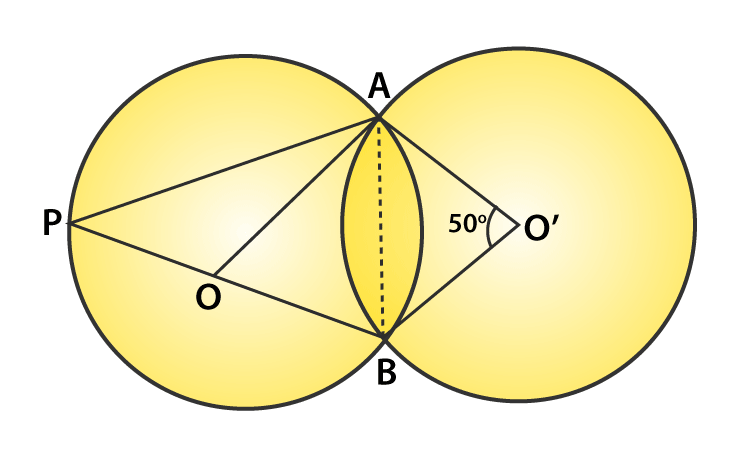Solution:

As we are given that, both the triangle are congruent which means their corresponding angles are equal.

Therefore, ∠AOB = AO’B = 50°

Now, by degree measure theorem, we have

∠APB = ∠AOB/2 = 250

Question 3: In figure, ABCD is a cyclic quadrilateral in which ∠BAD=75°, ∠ABD=58° and ∠ADC=77°, AC and BD intersect at P. Then, find ∠DPC.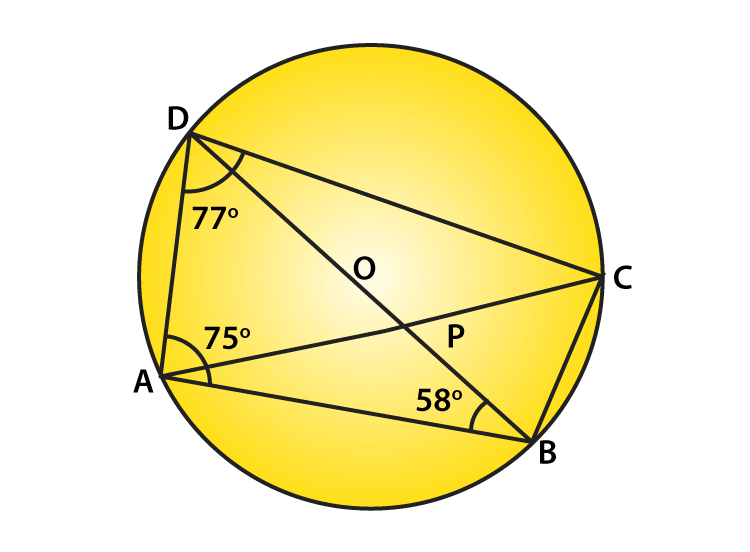Solution:

∠DBA = ∠DCA = 580 …(1)

[Angles in same segment]

ABCD is a cyclic quadrilateral :

Sum of opposite angles = 180 degrees

∠A +∠C = 1800

750 + ∠C = 1800

∠C = 1050

Again, ∠ACB + ∠ACD = 1050

∠ACB + 580 = 1050

or ∠ACB = 470 …(2)

Now, ∠ACB = ∠ADB = 470

[Angles in same segment]

Also, ∠D = 770 (Given)

Again From figure, ∠BDC + ∠ADB = 770

∠BDC + 470 = 770

∠BDC = 300

In triangle DPC

∠PDC + ∠DCP + ∠DPC = 1800

300 + 580 + ∠DPC = 1800

or ∠DPC = 92

Question 4: In figure, if ∠AOB = 80° and ∠ABC=30°, then find ∠CAO.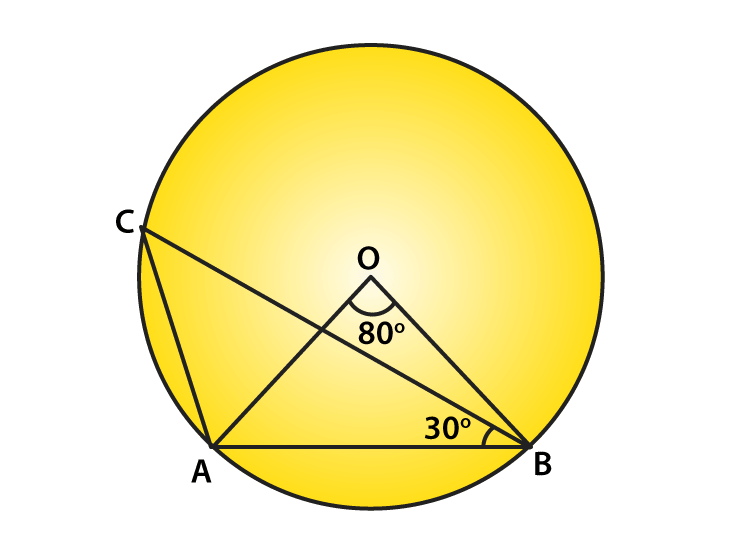Solution:

Given: ∠AOB = 800 and ∠ABC = 300

To find: ∠CAO

Join OC.

Central angle subtended by arc AC = ∠COA

then ∠COA = 2 x ∠ABC = 2 x 300 = 600 …(1)

In triangle OCA,

OC = OA

∠OCA = ∠CAO …(2)

[Angle opposite to equal sides]

In triangle COA,

∠OCA + ∠CAO + ∠COA = 1800

From (1) and (2), we get

2∠CAO + 600 = 1800

∠CAO = 600

## RD Sharma Solutions for Class 9 Maths Chapter 16 Circles Exercise VSAQs

RD Sharma Solutions Class 9 Maths Chapter 16 Circles Exercise VSAQs is based on the following topics and subtopics:

Circular Disc

Concentric Circles

Arc of a circle

Length of an arc

Central Angle

Minor Arc

Major Arc

Degree measure of an arc

Chord and segment of a circle

Some important results on Congruent arcs and chords